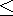Functions and CALL Routines

# PCTL Function

Returns the percentile that corresponds to the percentage.
 Category: Descriptive Statistics

## Syntax

 PCTL(percentage, value1<, value2, ...>)

### Arguments

n

is a digit from 1 to 5 which specifies the definition of the percentile to be computed.

 Default: definition 5
percentage

is a numeric constant, variable, or expression that specifies the percentile to be computed.

 Requirement: is numeric where, 0percentage100.
value

is a numeric variable, constant, or expression.

The PCTL function returns the percentile of the nonmissing values corresponding to the percentage. If percentage is missing, less than zero, or greater than 100, the PCTL function generates an error message.

Note:   The formula that is used in the PCTL function is the same formula that used in PROC UNIVARIATE. For more information, see SAS Elementary Statistics Procedures in Base SAS Procedures Guide.SAS Statements Results
```lower_quartile=PCTL(25,2,4,1,3);
put lower_quartile;```
`1.5`
```percentile_def2=PCTL2(25,2,4,1,3);
put percentile_def2;```
`1`
```lower_tertile=PCTL(100/3,2,4,1,3);
put lower_tertile;```
`2`
```percentile_def3=PCTL3(100/3,2,4,1,3);
put percentile_def3;```
`2`
```median=PCTL(50,2,4,1,3);
put median;```
`2.5`
```upper_tertile=PCTL(200/3,2,4,1,3);
put upper_tertile;```
`3`
```upper_quartile=PCTL(75,2,4,1,3);
put upper_quartile;```
`3.5`Previous Page | Next Page | Top of Page